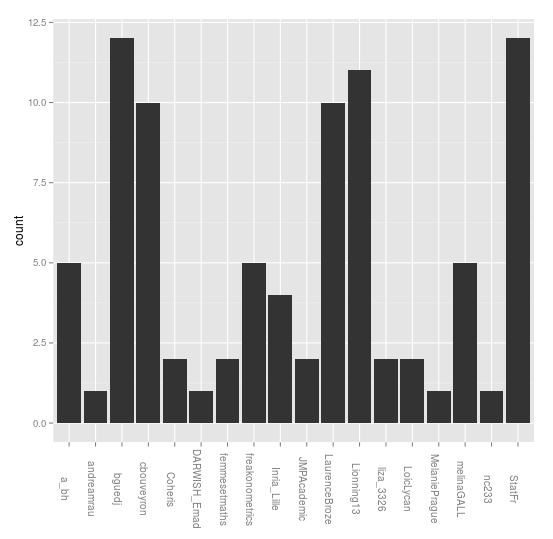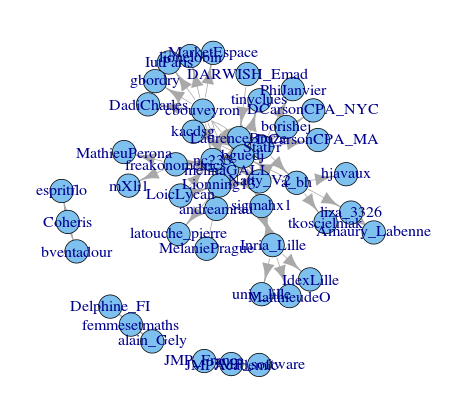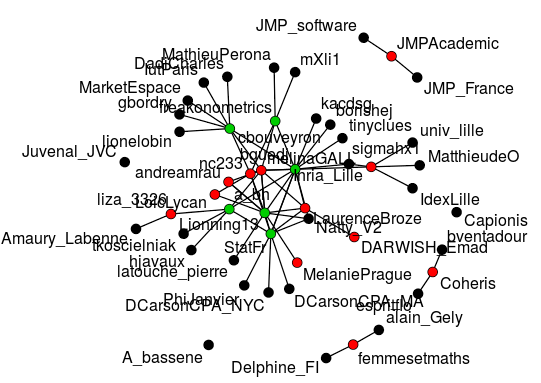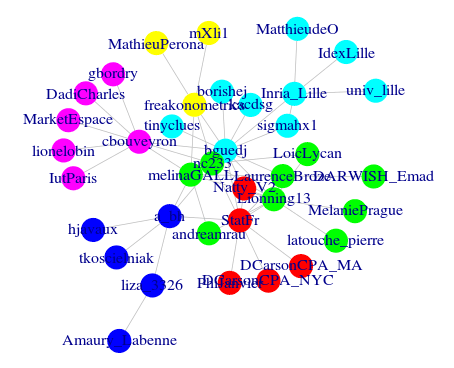Want to share your content on R-bloggers? click here if you have a blog, or here if you don't.

Disclamer: This is a joint post with Avner Bar-Hen, a.k.a. @a_bh, Benjamin Guedj, a.k.a. @bguedj and Nathalie Villa, a.k.a. @Natty_V2

Organised annually since 1970 by the French Society of Statistics (SFdS), the Journées de Statistique (JdS) are the most important scientific event of the French statistical community. More than 400 researchers, teachers and practitioners meet at each edition. In 2015, JDS took place in Lille, in France.

SFdS regularly tweets (with the account @Statfr) and for the first year a live-tweet was organized durind JdS. The Hashtag was #JDSLille. The aim of this post is a (brief) statistical analysis of the live-tweet.

Before starting, let us load some appropriate packages (and clean our R session)

```> rm(list=ls())
> graphics.off()
> library(tm)
> library(ggplot2)
> library(wordcloud)
> library(igraph)
> library(stringr)
> library(sna)```

Either you know how to scrap twitter data (see https://rhandbook.wordpress.com/tag/registertwitteroauth/ for example) and you can use the following code (after entering all the standard security parameters)

```> setup_twitter_oauth(.............)
> df <- do.call("rbind", lapply(tweet, as.data.frame))```

`> load(url("http://freakonometrics.free.fr/JDSLille.RData"))`

Now we have the data stored. Including the RT we found 219 tweets that represent 48 different users that posted atleast one tweet with the hashtag #JDSLille.

```> length(tweet)
 219```

The most active Twitter account was @bguedj, who initiates the tweet live. The second one is @Statfr the official account of SFdS.

```> counts=table(df\$screenName)
> subset(counts,counts>9)

bguedj  LaurenceBroze    Lionning13
46             12            24
melinaGALL          nc233        StatFr
12             15            26```

Let have a look at first to the RT. The graph below counts the number of RT per Twitter account

```> df\$text <- sapply(df\$text,
+ function(x) iconv(x,to='UTF-8'))
> trim <- function (x) sub('@','',x)
> df\$rt=sapply(df\$text,function(tweet)
trim(str_match(tweet,"^RT (@[[:alnum:]_]*)")))
> ggplot()+geom_bar(aes(x=na.omit(df\$rt)))+
theme(axis.text.x=element_text(angle=-90,size=10))+xlab(NULL)
```It is also possible to see when the tweet are posted. colors indicated the RT and grey indicated new posts.

```> ggplot()+geom_bar(aes(x=df\$created,
+ fill=df\$rt ))```Let’s have a look at the most common words within tweets.

```> myCorpus <- Corpus(VectorSource(df\$text))
> myCorpus <- tm_map(myCorpus, stripWhitespace)
> myCorpus <- tm_map(myCorpus, removePunctuation)
> myCorpus <- tm_map(myCorpus, removeNumbers)
> myStopwords <- c(stopwords("fr"), "RT", names(counts))
> myCorpus <- tm_map(myCorpus, removeWords, myStopwords)
> corpus.tdm <- TermDocumentMatrix(myCorpus, control = list(wordLengths = c(3,20)))
> findFreqTerms(corpus.tdm, lowfreq=10)
 "aléatoires"  "amphi"       "bigdata"
 "conférence"  "exposé"      "gala"
 "graphes"     "jdslille"    "journées"
 "les"         "lionning"    "matin"
 "présente"    "session"     "soirée"
 "statistique"```

Let us visualize the network

```> aretes = cbind(df\$rt,df\$screenName)
> aretes = aretes[apply(aretes,1,function(x)
+ sum(is.na(x))==0),]
> reseau = graph.data.frame(aretes,directed=T)
> plot(reseau)```As usual there is a big component (with 36 Twitter account) and few small ones (6 small clusters).Let’go further.

```> graphe = matrix(0,nrow=length(unique(c(df\$rt,
+ df\$screenName)))-1,ncol=length(unique(c(df\$rt,df\$screenName)))-1 )
> nom = unique(c(df\$rt,df\$screenName))
> rownames(graphe) = colnames(graphe) = nom[!is.na(nom)]
> for (i in 1:nrow(aretes)) graphe[aretes[i,1],
+ aretes[i,2]]=1
+ mode="undirected",weighted=TRUE,diag=FALSE)
> net.components <- clusters(net)
> net.components\$no
 7```

Let’s vizualize it

```> couleur = findInterval(apply(graphe,1,sum),
+ c(0,1,5,30))
> gplot(graphe,usearrows =FALSE,
+ displayisolates = TRUE, vertex.col=couleur,
+ label=colnames(graphe)```or if we zoom inBlack means that none of the post was RT (most of the time they only RT another post). Red is between 1 and 5 RT and green is for more than 5 RT.

Let’s look at the largest component. Density is around 8.1% and transitivity is about 23.7%. Therefore we have a strong preferential attachment.

```> net.lcc <- induced.subgraph(net,
+ net.components\$membership==
+ which.max(net.components\$csize))
> graph.density(net.lcc)
 0.08108108
> transitivity(net.lcc)
 0.2234043```

We can also use

```> net.clusters <- spinglass.community(net.lcc)
> table(net.clusters\$membership)

1 2 3 4 5 6 7
5 6 5 3 6 7 5
> V(net.lcc)\$community <- net.clusters\$membership
> plot(net.lcc, main="Communities",
+      vertex.frame.color=rainbow(max(
+net.clusters\$members))[net.clusters\$membership],
+      vertex.color=rainbow(max(
+net.clusters\$members))[net.clusters\$membership],
+      vertex.label=net.clusters\$names,
+      edge.color="grey")```or if we zoom inFor sure, many other results can be obtained from the dataset. Please feel free to use it. And all comments and feedback are welcome!

The interesting point with this code is that it is possible to adapt it for any conference! Use for instance the function

```conference = function(hash="#JDSLille",file=url("http://freakonometrics.free.fr/JDSLille.RData")){
if(is.na(file)){
df <- do.call("rbind", lapply(tweet, as.data.frame))
}
if(!is.na(file)){
}
counts=table(df\$screenName)
top_tw=rev(sort(subset(counts,counts>quantile(counts,.9))))
for(i in 1:length(top_tw)) cat("    @",names(top_tw)[i],": ",top_tw[i]," tweetsn",sep="")
df\$text <- sapply(df\$text,function(x) iconv(x,to='UTF-8'))
trim <- function (x) sub('@','',x)
df\$rt=sapply(df\$text,function(tweet) trim(str_match(tweet,"^RT (@[[:alnum:]_]*)")))
ggplot()+geom_bar(aes(x=na.omit(df\$rt)))+theme(axis.text.x=element_text(angle=-90,size=10))+xlab(NULL)
ggplot()+geom_bar(aes(x=df\$created,fill=df\$rt ))
myCorpus <- Corpus(VectorSource(df\$text))
myCorpus <- tm_map(myCorpus, stripWhitespace)
myCorpus <- tm_map(myCorpus, removePunctuation)
myCorpus <- tm_map(myCorpus, removeNumbers)
myStopwords <- c(stopwords("fr"), "RT", names(counts))
myCorpus <- tm_map(myCorpus, removeWords, myStopwords)
corpus.tdm <- TermDocumentMatrix(myCorpus, control = list(wordLengths = c(3,20)))
top_terms=findFreqTerms(corpus.tdm, lowfreq=10)
cat("Top Words -------------n")
for(i in 1:length(top_terms)) cat("    ",top_terms[i],"n",sep="")
aretes = cbind(df\$rt,df\$screenName)
aretes = aretes[apply(aretes,1,function(x)sum(is.na(x))==0),]
reseau = graph.data.frame(aretes,directed=T)
plot(reseau)
graphe = matrix(0,nrow=length(unique(c(df\$rt,df\$screenName)))-1,ncol=length(unique(c(df\$rt,df\$screenName)))-1 )
nom = unique(c(df\$rt,df\$screenName))
rownames(graphe) = colnames(graphe) = nom[!is.na(nom)]
for (i in 1:nrow(aretes)) graphe[aretes[i,1],aretes[i,2]]=1
net.components <- clusters(net)
net.components\$no
couleur = findInterval(apply(graphe,1,sum),c(0,1,5,30))
gplot(graphe,usearrows =FALSE,displayisolates = TRUE,vertex.col=couleur,label=colnames(graphe))
net.lcc <- induced.subgraph(net,net.components\$membership==
which.max(net.components\$csize))
graph.density(net.lcc)
transitivity(net.lcc)
net.clusters <- spinglass.community(net.lcc)
table(net.clusters\$membership)
V(net.lcc)\$community <- net.clusters\$membership
plot(net.lcc, main="Communities", vertex.frame.color=rainbow(max(net.clusters\$members))[net.clusters\$membership],
vertex.color=rainbow(max(net.clusters\$members))[net.clusters\$membership],
vertex.label=net.clusters\$names,
edge.color="grey")
}```

Note that the very last tweet related to the hashtag #JDSLille (during those five days) was

Indeed. That is a good idea ! See you next year for an update version of the statistical analysis of the tweet-live, on #JDSMontpellier.